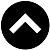-->

Type something and hit enter

# 我国的巴菲特指标2017年：GDP = 1.3兆令吉，马股总市值 = 1.9兆令吉，指标 = 147%
2018年：GDP = 1.36兆令吉，马股总市值 = 1.7兆令吉，指标 = 125%
2019年：GDP = 1.42兆令吉，马股总市值 = 1.7兆令吉，指标 = 120%
2019年7月至2020年6月：GDP = 1.36兆令吉，马股总市值 = 1.5兆令吉，指标 = 116%

2017年：GDP = 1.3兆令吉，M2 = 1.73兆令吉，比率 = 0.75
2018年：GDP = 1.36兆令吉，M2 = 1.88兆令吉，比率 = 0.72
2019年：GDP = 1.42兆令吉，M2 = 1.95兆令吉，比率 = 0.73
2019年7月至2020年6月：GDP = 1.36兆令吉，M2 = 2.01兆令吉，比率 = 0.68

https://investalone.blogspot.com/2020/08/blog-post_16.html